• 【数学知识】函数与复合函数编程实现1、函数定义多项式指数函数与对数函数正弦函数2、复合函数 本博客适合高中学生入门编程知识学习，从高中的数学概念转换到其python实现，提高自身对编程的学习兴趣。 import numpy...
【数学知识】函数与复合函数编程实现1、函数定义多项式指数函数与对数函数正弦函数2、复合函数
本博客适合高中学生入门编程知识学习，从高中的数学概念转换到其python实现，提高自身对编程的学习兴趣。
import numpy as np
import matplotlib.pyplot as plt
%matplotlib inline
# 在jupyter notebook显示图片

1、函数定义
我们可以将函数(functions)想象成一台机器f ，每当我们向机器提供输入x，这台机器便会产生输出y。
这台机器所能接受的所有输入x的集合称为定义域(domain)，其所有可能输出y的集合称为值域(range)。函数的定义域和值域有着非常重要的意义，如果我们知道一个函数的定义域，便不会将不合适的输入丢给函数；知道函数的值域，便能判断一个值是否可能是这个函数所输出的。
多项式
# 多项式
# 初中：一元一次函数 y = k*x+b
def f1(x):
y = 2*x+3  # k=2, b=3
return y


x = 100
print(f1(100))

203

三次多项式：
$f(x)=x^3-5x^2+9$
# 三次多项式
def f3(x):
return x**3 - 5*x**2 + 9  # *：乘号 **：求次方

# 画图
x = np.linspace(-100, 100, num = 1000)
y = f3(x)
plt.plot(x,y)

结果如下：# 5次多项式
def f5(x):
return 5*x**5+4*x**3+x**3 - 5*x**2 + 9  # *：乘号 **：求次方

y = f5(x)
plt.plot(x,y)指数函数与对数函数
两个常用的常数：
欧拉常数e
$e = 2.718281828459045$
圆周率pi
$\pi = 3.141592653589793$
# 欧拉常数e
print(np.e)  # e=2.71828
# pi
print(np.pi)  # pi=3.1415926

# 指数函数
def exp(x):
y = np.e**x  # np.e 就是欧拉常数
return y  # y>0

y = exp(x)
plt.plot(x,y)

结果如下：print(exp(0))

1.0

print(exp(-10000))

0.0

$e^x$的本质：
$e^x={x^0}/{0!}+{x^1}/{1!}+{x^2}/{2!}+{x^3}/{3!}+...+{x^n}/{n!}$
对数函数是指数函数的反函数
如果 $y=e^x$
那么 $x=ln(y)$,  y>0
x = np.linspace(0.0001,100,1000,endpoint = False)
y1 = np.log2(x)  # 以2为底
y2 = np.log(x)   # 以e为底
y3 = np.log10(x)  # 以10为底
plt.plot(x,y1,'red',x,y2,'yellow',x,y3,'blue')正弦函数
正弦函数:
$y = sin(x)$
以及它的变形
$y = Asin({\omega}x+\theta)$
x = np.linspace(-10, 10, num = 1000)
y = np.sin(x)
plt.plot(x,y)

结果如下：余弦函数:
$y = cos(x)=sin(x+{\pi}/2)$
以及它的变形
$y = Acos({\omega}x+\theta)$
y1 = np.sin(x)   # sin(x)
y2 = np.cos(x)  # cos(x)
plt.plot(x,y1,'red',x,y2,'blue')

下图中红色为sin(x)，蓝色为cos(x)：2、复合函数
函数$f(x)$和$g(x)$的复合$fg(x)$:，可以理解为首先将输入$x$给函数$g(x)$获得输出y1后将其进而输入给函数$f(x)$，最终获得结果$y$。例如：
$f(x) = 1/x$
$g(x) = e^{-x}+1$
$fg(x) = f(g(x)) = 1/(1+e^{-x})$
这个复合函数实际上是$sigmoid(x)$
链式法则是微积分中的求导法则，用于求一个复合函数的导数
采用复合函数表示十分复杂的函数，有利于我们进行求导运算！
同时，在编程过程中，采用复合函数，将一个复杂的问题分解为若干个阶段的函数，有利于编程调试，容易发现逻辑错误！
def f(x):
y = 1/x
return y

def g(x):
y = np.exp(-x)+1
return y

# 这是一个复合函数
def sigmoid(x):
return f(g(x))

x = 1
print(sigmoid(x))

0.7310585786300049

x = np.linspace(-10, 10, num = 10000)
y = sigmoid(x)
plt.plot(x,y)另一个常用的复合函数：$softplus(x)$
$f(x) = log(x)$
$g(x) = 1+e^x$
$fg(x) = f(g(x)) = log(1+e^x)$
def f(x):
y = np.log(x)
return y

def g(x):
y = np.exp(x)+1
return y

# 这是一个复合函数
def softplus(x):
return f(g(x))

x = 1
print(softplus(x))

1.3132616875182228

x = np.linspace(-10, 10, num = 10000)
y = softplus(x)
plt.plot(x,y)【作者简介】陈艺荣，男，目前在华南理工大学电子与信息学院广东省人体数据科学工程技术研究中心攻读博士，担任IEEE Access、IEEE Photonics Journal的审稿人。两次获得美国大学生数学建模竞赛(MCM)一等奖，获得2017年全国大学生数学建模竞赛(广东赛区)一等奖、2018年广东省大学生电子设计竞赛一等奖等科技竞赛奖项，主持一项2017-2019年国家级大学生创新训练项目获得优秀结题，参与两项广东大学生科技创新培育专项资金、一项2018-2019年国家级大学生创新训练项目获得良好结题，发表SCI论文4篇，授权实用新型专利8项，受理发明专利13项。
我的主页
我的Github
我的CSDN博客


展开全文python 数学基础
• 本文讨论了一类指数函数组合在复合意义下的分解。这一类指数函数组合中作为指数的多项式可以具有相同的次数。
• 分解
分解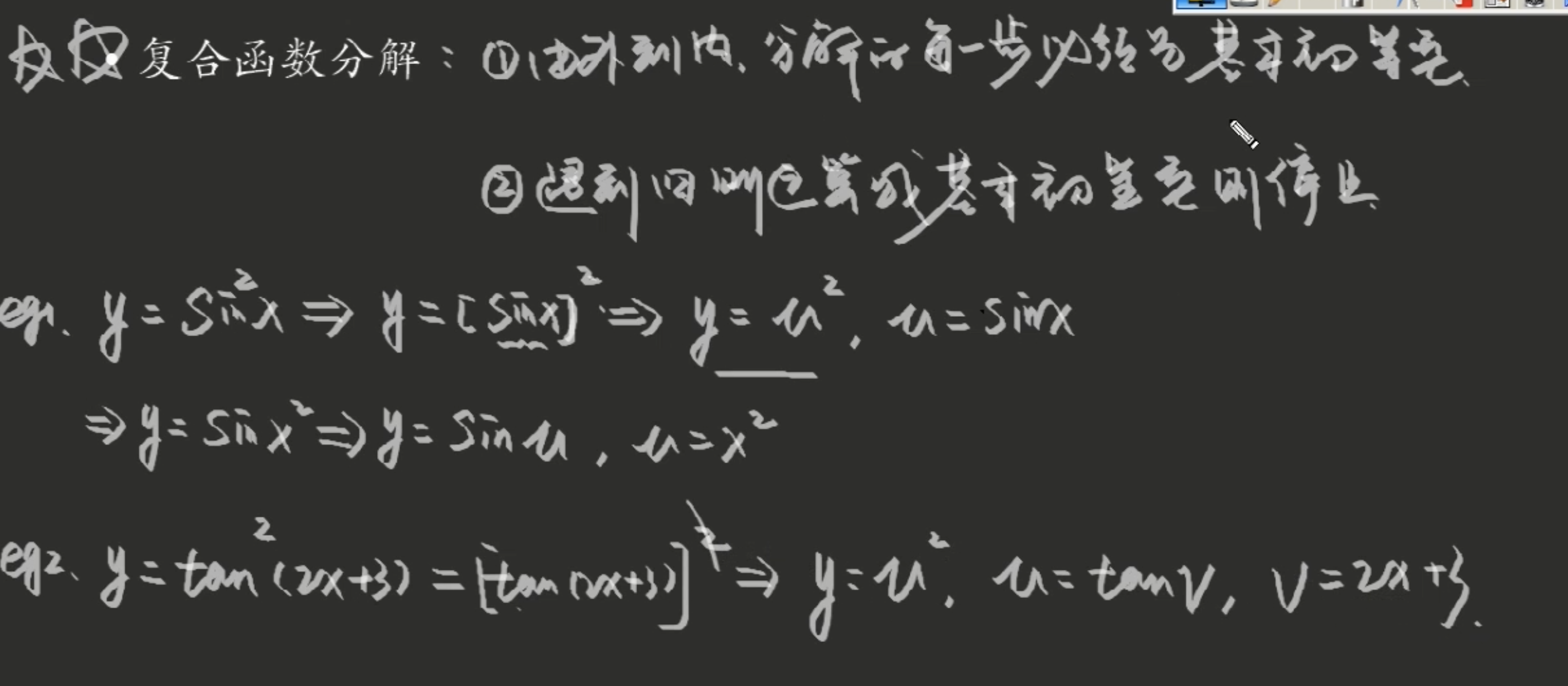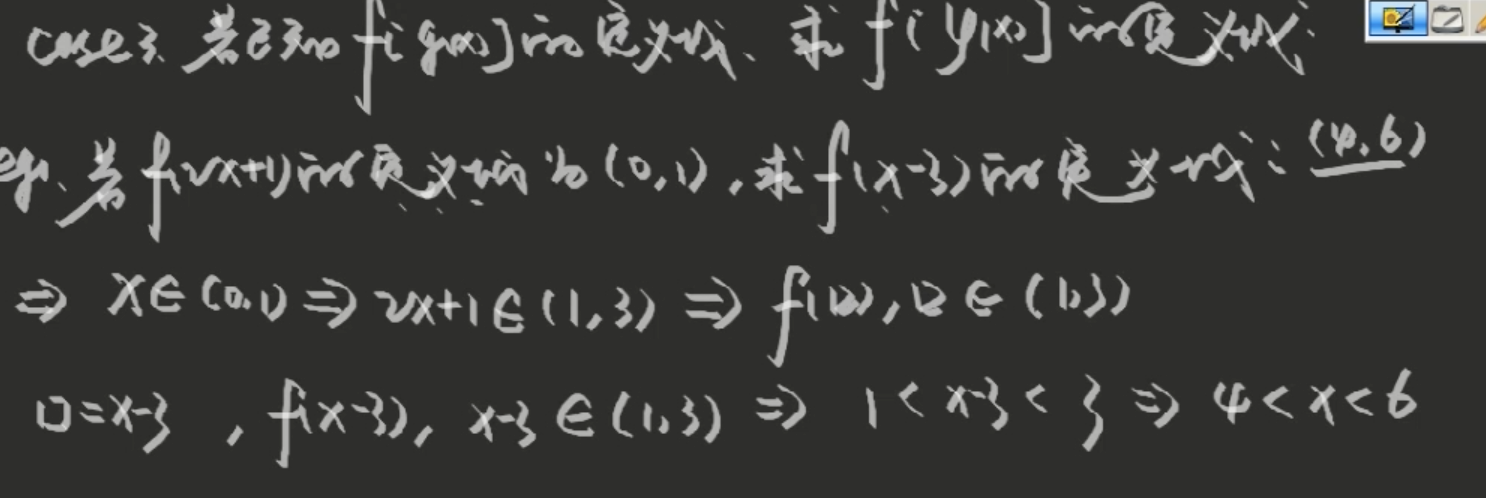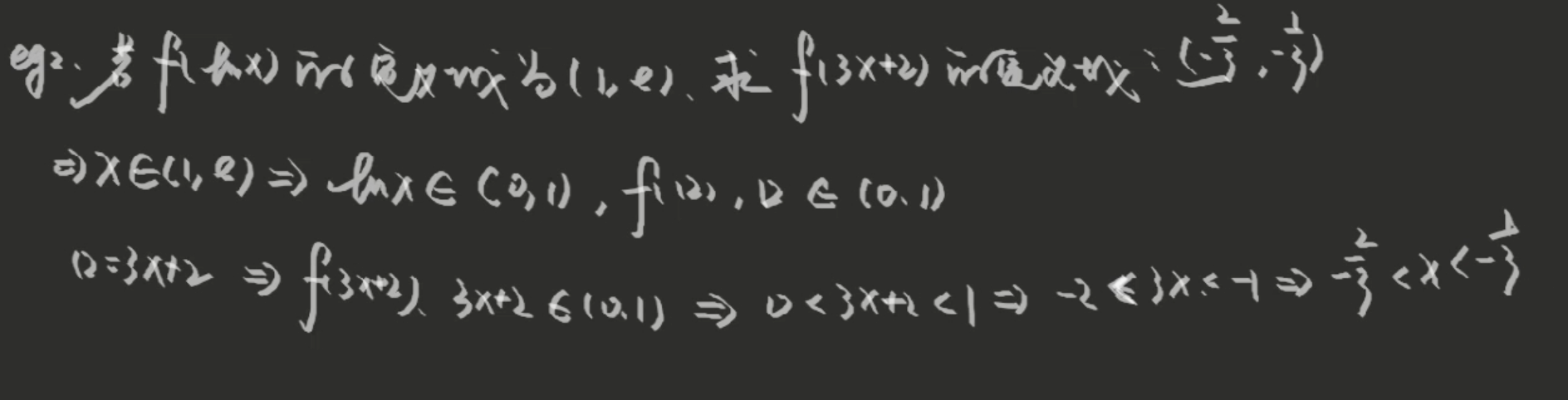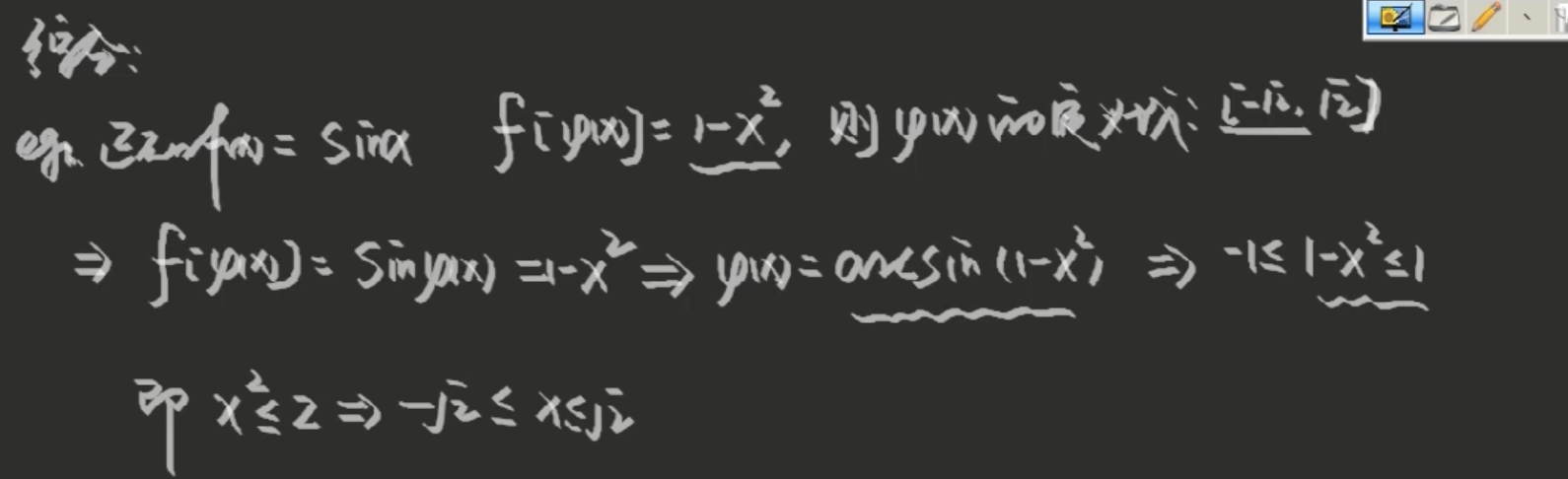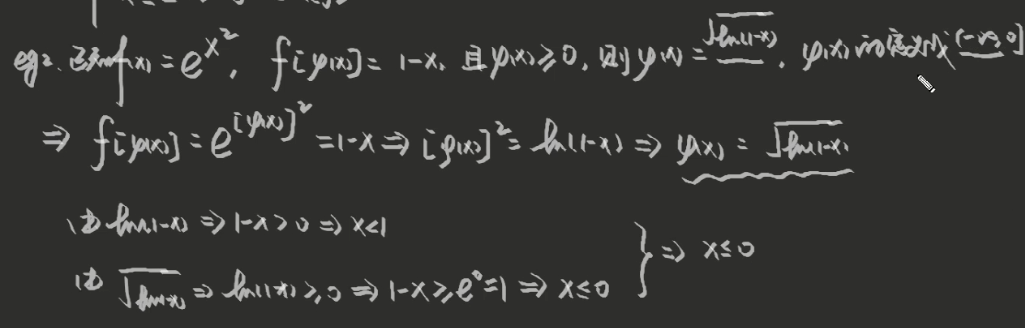展开全文线性代数
• 考虑下面函数的导数 y=(x3+2)5(1)\begin{equation} y=(x^3+2)^5\tag1 \end{equation}根据目前现有的工具，我们可以利用二项式定理将函数展开成多项式进行求导。 y=x15+10x12+40x9+80x6+80x3+32.(2)\begin{equation...
考虑下面函数的导数
y=(x3+2)5(1)\begin{equation}
y=(x^3+2)^5\tag1
\end{equation}

根据目前现有的工具，我们可以利用二项式定理将函数展开成多项式进行求导。
y=x15+10x12+40x9+80x6+80x3+32.(2)\begin{equation}
y=x^{15}+10x^{12}+40x^9+80x^6+80x^3+32.\tag2
\end{equation}

然后可以立马求出导数
dydx=15x14+120x11+360x8+480x5+240x2.(3)\begin{equation}
\frac{dy}{dx}=15x^{14}+120x^{11}+360x^8+480x^5+240x^2.\tag3
\end{equation}

展开比较讨人厌但是不难。然而，很少人愿意对函数y=(x3+2)100$y=(x^3+2)^{100}$进行同样的操作。最好能有一种链式法则，可以对每个函数的求导都变得容易。

出于此目的，理解函数(1)的结构非常重要。通过引入辅助变量u=x3+2$u=x^3+2$来实现此目的，这样的话(1)式就可以分解成两个简单的部分：
y=u2u=x3+2(4)\begin{equation}
\end{equation}

我们将u$u$的表达式带入y=u5$y=u^5$重新构成(1)。这样的函数叫做复合函数，或函数的函数。一般来说，假设y$y$是u$u$的函数，而u$u$是x$x$的函数，
y=f(u)u=f(x)(5)\begin{equation}
\end{equation}

对应的复合函数就是
y=f(g(x))(6)\begin{equation}
y=f(g(x))\tag6
\end{equation}

假定我们现在有一个(6)那样的复合函数，我们希望知道如何将它分解成(5)那样容易求导的形式来计算它的导数。这就是链式法则的内容。

7 链式法则：在上面描述的环境下，我们有
dydx=dydu⋅dudx.(7)\begin{equation}
\frac{dy}{dx}=\frac{dy}{du}\cdot\frac{du}{dx}.\tag7
\end{equation}

如我们所见，链式法则有点代数的特点；因为莱布尼兹的导数符号表明两个分式中的du$du$可以消掉，所以这个公式很好记忆。如果我们将导数看作变化率的话，直观上也很容易理解：

如果y$y$的变化速度是u$u$的a$a$倍，u$u$的变化速度是x$x$的b$b$倍，那么y$y$的变化速度是x$x$的ab$ab$倍。

或者用日常用语来说，如果车的速度是自行车的两倍，自行车的速度是步行的四倍，那么车的速度是步行的2⋅4=8$2\cdot 4=8$倍。

在进行链式法则证明之前，让我们先看看它是如何应用刚才讨论的问题上，(1)是给定的函数，(4)是它的分解形式。根据(7)
dydx=dydu⋅dudx=5u4⋅3x2=15x2(x3+2)4.(8)\begin{equation}
\frac{dy}{dx}=\frac{dy}{du}\cdot\frac{du}{dx}=5u^4\cdot 3x^2=15x^2(x^3+2)^4.\tag8
\end{equation}

最后一步用x3+2$x^3+2$替换辅助变量u$u$。这个结果跟(3)一样，直接看似乎不太明显，但是等价是很容易建立起来的。更进一步，y=(x3+2)100$y=(x^3+2)^{100}$的导数可以用相同的方式很容易就计算出来：
y=u100u=x3+2

利用(7)式得到
dydx=dydududx==100u99⋅3x2300x2(x3+2)99.\begin{eqnarray*}
\frac{dy}{dx}=\frac{dy}{du}\frac{du}{dx}
&=&100u^{99}\cdot 3x^2\\
&=&300x^2(x^3+2)^{99}.
\end{eqnarray*}

正如这些例子展示的那样，链式法则是个强大的工具。

跟之前一样，我们用自变量的变化量Δx$\Delta x$来证明(7)式；对于变量u$u$产生的变化量是Δu$\Delta u$，那么对于y$y$就是Δy$\Delta y$。我们知道可导隐含着连续，所以Δx→0$\Delta x\to 0$时Δu→0$\Delta u\to 0$。看三个导数的定义，我们现在试图将它们连接起来
dydx=limΔx→0ΔyΔx,dydu=limΔu→0ΔyΔu,dudx=limΔx→0ΔuΔx,(9)\begin{equation}
\frac{dy}{dx}=\lim_{\Delta x\to 0}\frac{\Delta y}{\Delta x},\quad \frac{dy}{du}=\lim_{\Delta u\to 0}\frac{\Delta y}{\Delta u},\quad \frac{du}{dx}=\lim_{\Delta x\to 0}\frac{\Delta u}{\Delta x},\tag9
\end{equation}

那么很自然的我们尝试用简单的代数知识来完成证明：
ΔyΔx=ΔyΔu⋅ΔuΔx,(10)\begin{equation}
\frac{\Delta y}{\Delta x}=\frac{\Delta y}{\Delta u}\cdot \frac{\Delta u}{\Delta x}, \tag{10}
\end{equation}

所以
dydx=limΔx→0ΔyΔx=limΔx→0ΔyΔu⋅ΔuΔx=[limΔx→0ΔyΔu][limΔx→0ΔuΔx]=[limΔu→0ΔyΔu][limΔx→0ΔuΔx]=dydu⋅dudx(11)\begin{align}
\frac{dy}{dx}
&=\lim_{\Delta x\to 0}\frac{\Delta y}{\Delta x}=\lim_{\Delta x\to 0}\frac{\Delta y}{\Delta u}\cdot \frac{\Delta u}{\Delta x}=\left[\lim_{\Delta x\to 0}\frac{\Delta y}{\Delta u}\right]\left[\lim_{\Delta x\to 0}\frac{\Delta u}{\Delta x}\right]\notag\\
&=\left[\lim_{\Delta u\to 0}\frac{\Delta y}{\Delta u}\right]\left[\lim_{\Delta x\to 0}\frac{\Delta u}{\Delta x}\right]=\frac{dy}{du}\cdot \frac{du}{dx}\tag{11}
\end{align}

这个推理几乎完全正确，但是不绝对。难点在于除数为零。根据(9)中的定义来计算dy/dx$dy/dx$时，增量Δx$\Delta x$趋近于0但不等于零时，公式才是有意义的。另一方面，Δx$\Delta x$可能对u$u$不产生影响，即Δu=0$\Delta u= 0$，这样的话(10)(11)就不成立。这个缺陷用巧妙的数学诡计就能填补。

链式法则对所有级别的求导包括最简单的都是必不可少的。在寻找(x3+2)5$(x^3+2)^5$和(x3+2)100$(x^3+2)^{100}$导数的联系中给出了说明。通用的法则可以表示为如下的形式
ddx()n=n( )n−1ddx( )
\frac{d}{dx}()^n=n(\ )^{n-1}\frac{d}{dx}(\ )

任何对x$x$可导的函数都能放入括号内。如果给定一个关于u$u$的函数，那么公式可以写成如下形式。
8 幂法则：
ddxun=nun−1dudx.(12)\begin{equation}
\frac{d}{dx}u^n=nu^{n-1}\frac{du}{dx}.\tag{12}
\end{equation}

目前我们知道指数n$n$可以为正，可以为负，也可以为零。之后我们将会看到它也可以为分数。

例1：对y=(3x4+1)7$y=(3x^4+1)^7$求导，应用公式(12)
dydx=7(3x4+1)6ddx(3x4+1)=7(3x4+1)6⋅12x3.
\frac{dy}{dx}=7(3x^4+1)^6\frac{d}{dx}(3x^4+1)=7(3x^4+1)^6\cdot 12x^3.

而对y=[(3x4+1)7+1]5$y=[(3x^4+1)^7+1]^5$求导，我们需要连续用两次(12)：
dydx===5[(3x4+1)7+1]4ddx[(3x4+1)7+1]5[(3x4+1)7+1]4⋅7(3x4+1)6ddx(3x4+1)5[(3x4+1)7+1]4⋅7(3x4+1)6⋅12x3.\begin{eqnarray*}
\frac{dy}{dx}
&=&5[(3x^4+1)^7+1]^4\frac{d}{dx}[(3x^4+1)^7+1]\\
&=&5[(3x^4+1)^7+1]^4\cdot 7(3x^4+1)^6\frac{d}{dx}(3x^4+1)\\
&=&5[(3x^4+1)^7+1]^4\cdot 7(3x^4+1)^6\cdot 12x^3.
\end{eqnarray*}

对这个过程熟悉后，经常跳过其中的几步直接写出来。目前为了更清晰，我们将计算的每一步都写出来。

例2：如果y=[(1−2x)/(1+2x)]4$y=[(1-2x)/(1+2x)]^4$，那么根据(12)和除法法则
dydx===4((1−2x)1+2x)ddx(1−2x1+2x)4((1−2x)1+2x)⋅(1+2x)(−2)−(1−2x)(2)(1+2x)2−16(1−2x)3(1+2x)5.\begin{eqnarray*}
\frac{dy}{dx}
&=&4\left(\frac{(1-2x)}{1+2x}\right)\frac{d}{dx}\left(\frac{1-2x}{1+2x}\right)\\
&=&4\left(\frac{(1-2x)}{1+2x}\right)\cdot \frac{(1+2x)(-2)-(1-2x)(2)}{(1+2x)^2}\\
&=&\frac{-16(1-2x)^3}{(1+2x)^5}.
\end{eqnarray*}

例3：如果y=(x2−1)3(x2+1)−2$y=(x^2-1)^3(x^2+1)^{-2}$，那么根据(12)和乘法法则
dydx==(x2−1)3ddx(x2+1)−2+(x2+1)−2ddx(x2−1)3(x2−1)3⋅(−2)(x2+1)−3(2x)+(x2+1)−2⋅3(x2−1)2(2x).\begin{eqnarray*}
\frac{dy}{dx}
&=&(x^2-1)^3\frac{d}{dx}(x^2+1)^{-2}+(x^2+1)^{-2}\frac{d}{dx}(x^2-1)^3\\
&=&(x^2-1)^3\cdot (-2)(x^2+1)^{-3}(2x)+(x^2+1)^{-2}\cdot 3(x^2-1)^2(2x).
\end{eqnarray*}

为了化简，我们提出公因子2x(x2−1)2$2x(x^2-1)^2$，去掉负指数，并通分：
dydx==2x(x2−1)2[−2(x2−1)(x2+1)3+3(x2+1)2]2x(x2−1)2[−2(x2−1)+3(x2+1)(x2+1)3]=2x(x2−1)(x2+5)(x2+1)3.\begin{eqnarray*}
\frac{dy}{dx}
&=&2x(x^2-1)^2\left[\frac{-2(x^2-1)}{(x^2+1)^3}+\frac{3}{(x^2+1)^2}\right]\\
&=&2x(x^2-1)^2\left[\frac{-2(x^2-1)+3(x^2+1)}{(x^2+1)^3}\right]=\frac{2x(x^2-1)(x^2+5)}{(x^2+1)^3}.
\end{eqnarray*}

之后我们将在许多科学和几何的具体问题上将求导作为工具，那时候将清楚的看到将我们计算的导数化简成最简形式是非常值得的。

还有一些结束语应给给出。我们一直没有解释为什么链式法则是合适的。理由就是，(7)式中我们处理的三个变量y,u,x$y,u,x$都是互相联系的，每一个依赖于下一个。这个关系可以写成

y$y$依赖于u$u$依赖于x$x$.
公式
dydx=dydu⋅dudx
\frac{dy}{dx}=\frac{dy}{du}\cdot \frac{du}{dx}

告诉我们如何计算第一个变量相对于最后一个变量的导数，那就是考虑链中的各个部分。这个公式很容易扩展到多变量的情况。例如，如果x$x$依赖于z$z$，那么
dydz=dydu⋅dudx⋅dxdz
\frac{dy}{dz}=\frac{dy}{du}\cdot \frac{du}{dx}\cdot \frac{dx}{dz}

如果z$z$依赖于w$w$，那么
dydw=dydu⋅dudx⋅dxdz⋅dzdw
\frac{dy}{dw}=\frac{dy}{du}\cdot \frac{du}{dx}\cdot \frac{dx}{dz}\cdot\frac{dz}{dw}

等等。每个新变量链中就增加一个新的链接，公式中就增加一个新的导数。
展开全文链式法则
• 目录数据库系统原理-函数依赖关系模式分解第一范式如何处理非原子值原子性关系数据库设计中易犯的错误模式分解无损连接分解优化关系模式的步骤函数依赖函数依赖定义函数依赖的使用函数依赖集的闭包Armstrong公理...
数据库系统原理-函数依赖和关系模式分解
目录数据库系统原理-函数依赖和关系模式分解第一范式如何处理非原子值原子性关系数据库设计中易犯的错误模式分解无损连接分解优化关系模式的步骤函数依赖函数依赖定义函数依赖的使用函数依赖集的闭包Armstrong公理Armstrong公理二级定律闭包计算算法属性集的闭包属性闭包的用法正则覆盖无关属性检测属性是否无关正则覆盖规范化-模式分解分解验证算法模式分解总结规范化-简单版图示法求候选键主属性和非主属性第一范式（1NF）第二范式（2NF）第三范式（3NF）BC范式（BCNF）模式分解-保持函数依赖分解模式分解-无损分解
学习本章相关理论知识可以为优化我们设计的数据库模型
第一范式
如果某个域中元素被认为是不可分的，则这个域称为是原子的
非原子域的例子：

复合属性：名字集合
多值属性：电话号码
复杂数据类型：面向对象的

如果关系模式R的所有属性的域都是原子的，则R称为属于第一范式
（1NF）
非原子值存储复杂并易导致数据冗余，在数据库系统中我们创建的表基本都属于第一范式
如何处理非原子值

对于组合属性：让每个子属性本身成为一个单独的属性
对于多值属性：为多值集合中的每个项创建一条元组（再建表）

原子性
原子性实际上是由域元素在数据库中如何被使用决定的
例如，字符串通常会被认为是不可分割的，假设学生被分配这样的标识号：CS0012或SE1127，如果前两个字母表示系，后四位数字表示学生在该系内的唯一号码，则这样的标识号不是原子的（字符串+数字）
当采用这种标识号时，是不可取的。因为这需要额外的编程，而且信息是在应用程序中而不是在数据库中编码
关系数据库设计中易犯的错误
关系数据库设计要求我们找到一个好的关系模式集合。一个坏的
设计可能导致以下问题

数据冗余
插入、删除、修改异常

假设，我们用以下模式代替instructor模式和department模式：
inst_dept(ID, name, salary, dept_name, building, budget)

这样的关系模式存在以下问题

数据冗余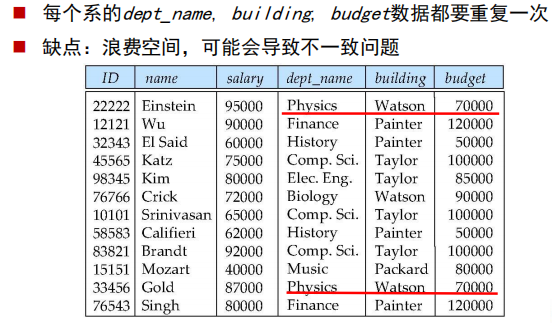更新异常

更新复杂，容易导致不一致问题。例，修改dept_name，很多相关元组都需要修改

插入/删除异常

使用空值(null)：存储一个不知道所在系的教师信息，可以使用空值表示dept_name, building, budget数据，但是空值难以处理
模式分解
例：可以将关系模式(A,B,C,D)分解为：(A,B)和(B,C,D)，或(A,C,D)和
(A,B,D)，或(A,B,C)和(C,D)，或(A,B)、(B,C)和(C,D)，或 (A,D)和
(B,C,D)
例：将关系模式inst_dept分解为：
― *instructor*(*ID,name,dept_name,salary*)

― *department*(*dept_name,building,budget*)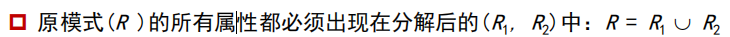无损连接分解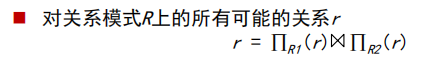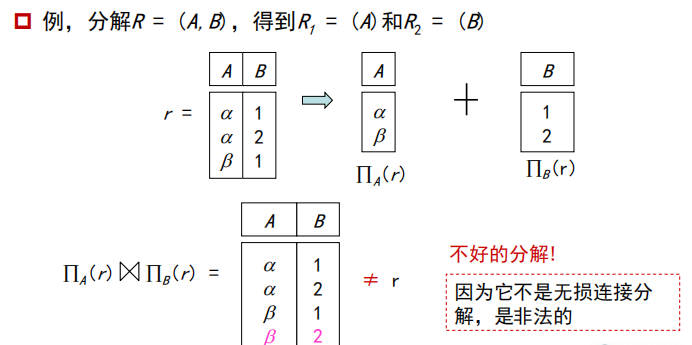优化关系模式的步骤
判断关系模式好坏—>若是后者—>关系模式分解解成模式集合{R1**, R**2, …, Rn}使得：每个关系模式都是“好的形式”且分解是无损连接分解
我们优化关系模式的理论基于

函数依赖（ functional dependencies ）
多值依赖（ multivalued dependencies ）

函数依赖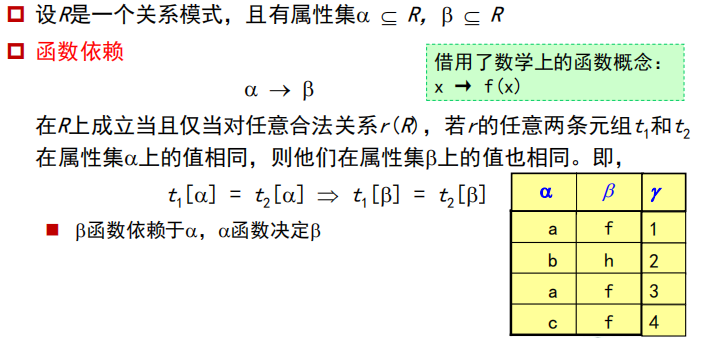函数依赖定义
一种完整性约束，表示特定的属性值之间的关系，可以用来判断模式规范化和建议改进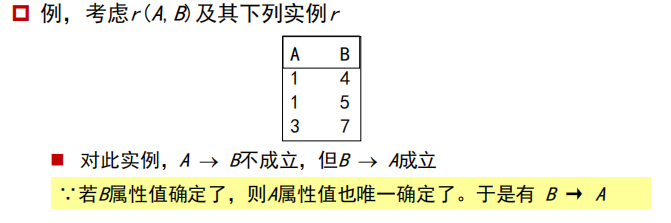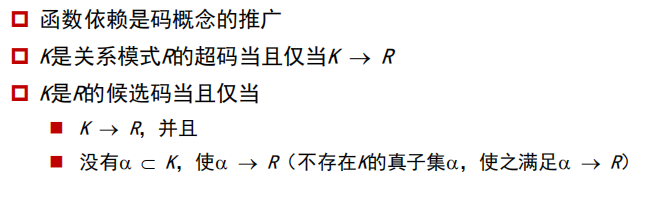这里解释以下，候选码是最小超码
函数依赖使我们可以表达用超码无法表达的约束，考虑模式
inst_dept(ID,name,salary,dept_name,building,budget)
/*budget表示预算*/
/*building表示部门地点*/

我们期望下列函数依赖成立：
dept_name -->building
ID --> building

而不期望下列函数依赖成立：
dept_name --> salary

函数依赖的使用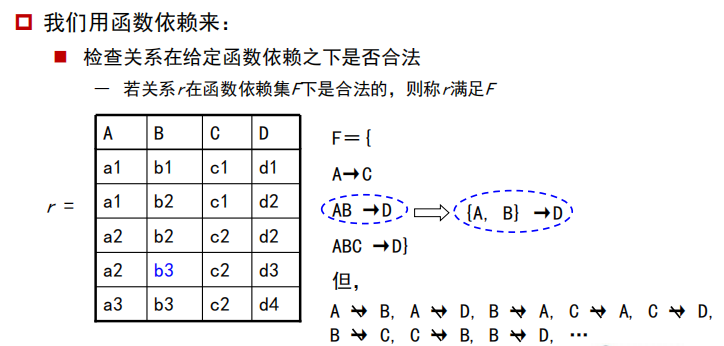函数依赖集：多条函数依赖（A->B）的集合
可以这么理解，A列相同的值对应C列相同的值如a1->c1 a2->c2…

[外链图片转存失败,源站可能有防盗链机制,建议将图片保存下来直接上传(img-mfD8YTDp-1574005601262)(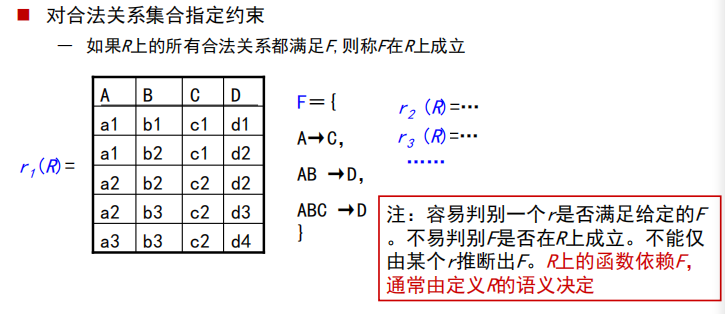)]
R是关系模式
r是关系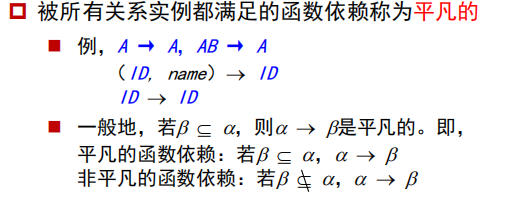函数依赖集的闭包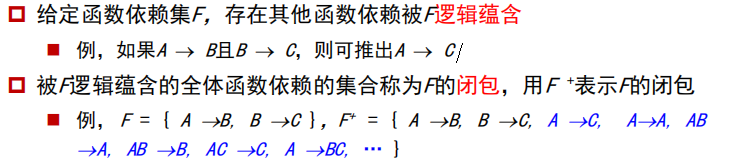Armstrong公理
利用Armstrong公理来找出函数依赖集的闭包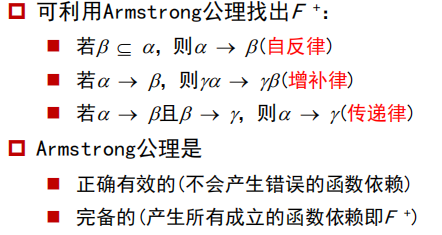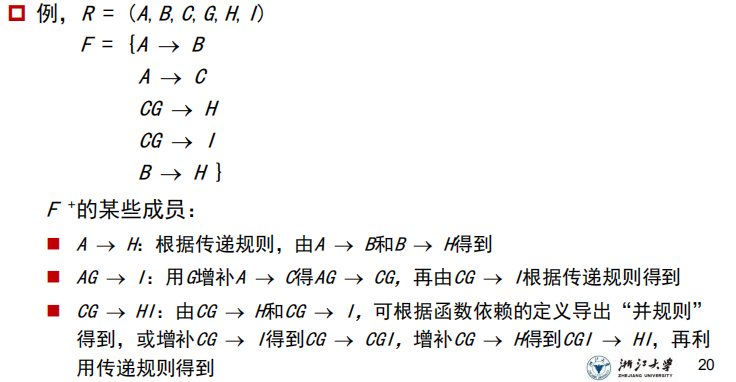Armstrong公理二级定律闭包计算算法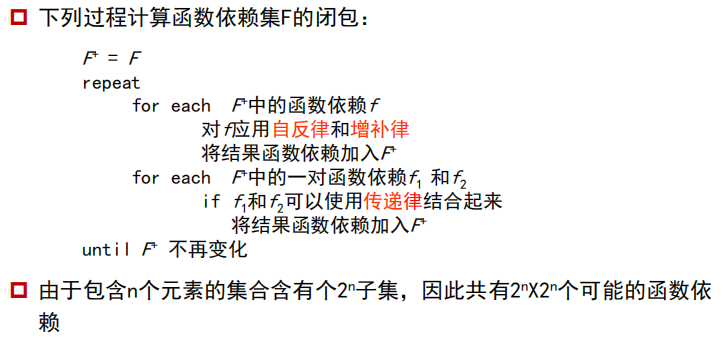属性集的闭包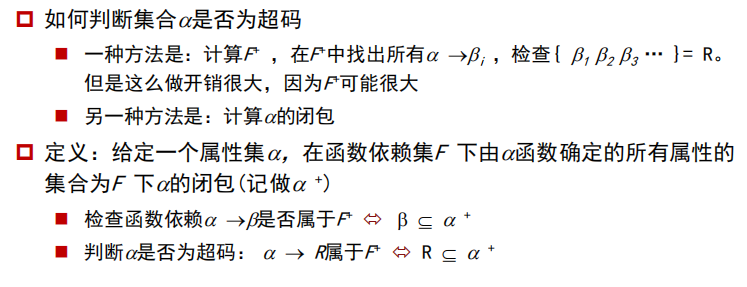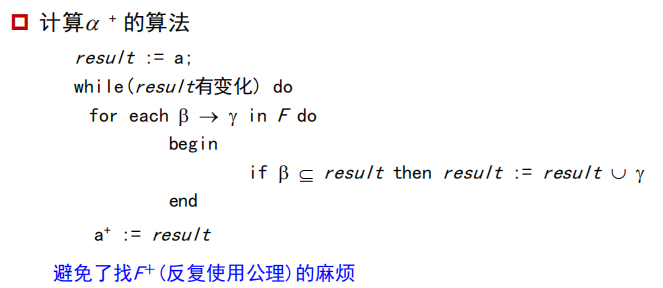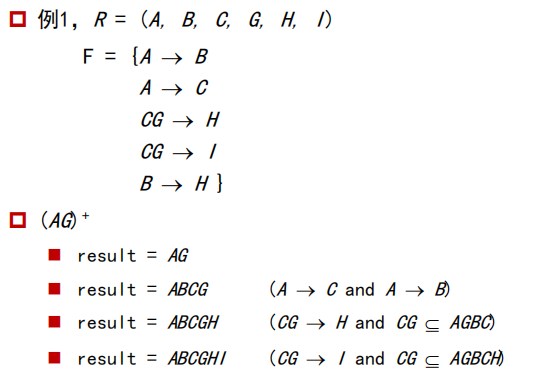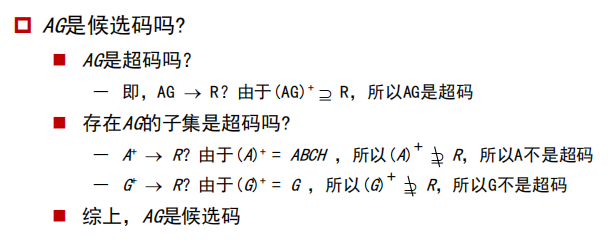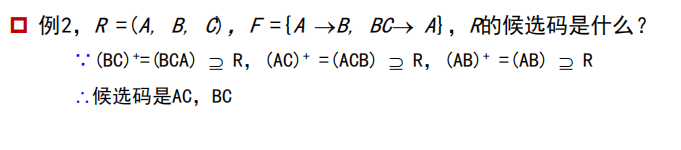属性闭包的用法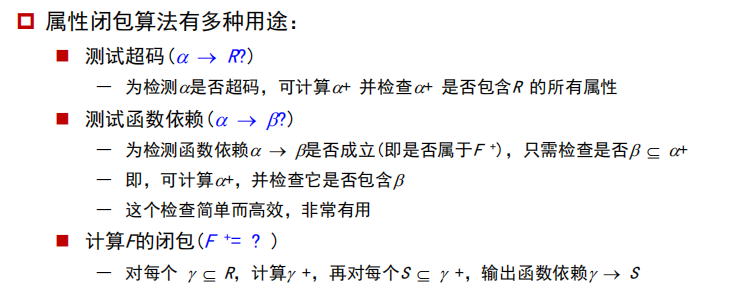正则覆盖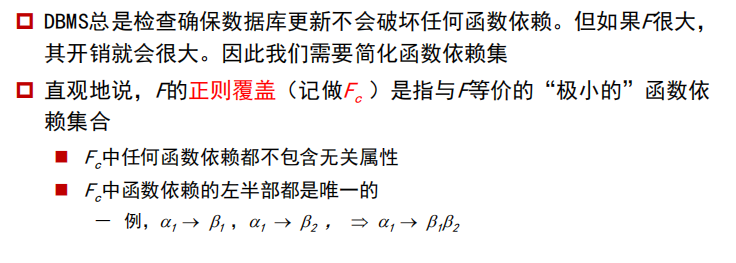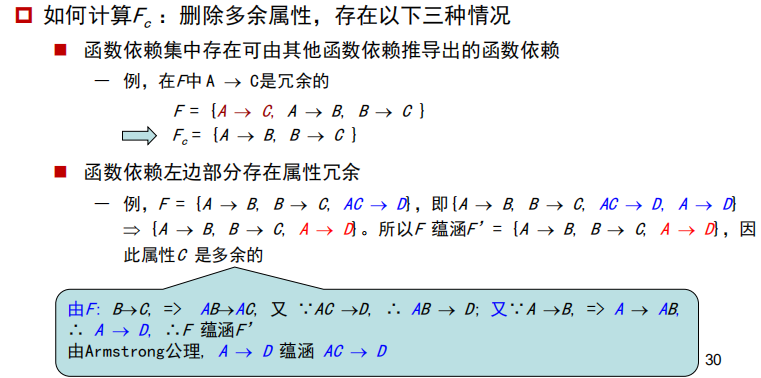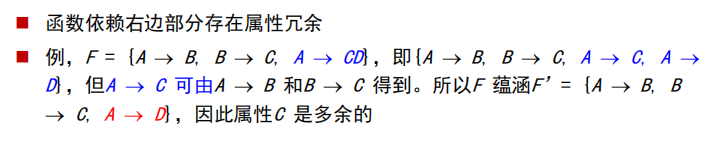无关属性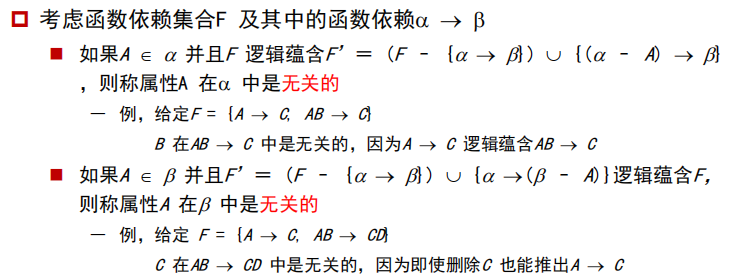检测属性是否无关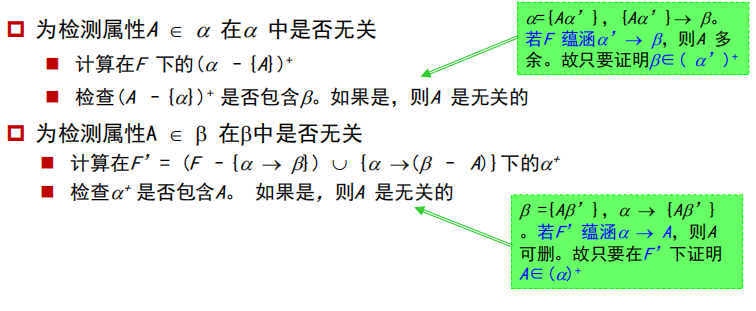正则覆盖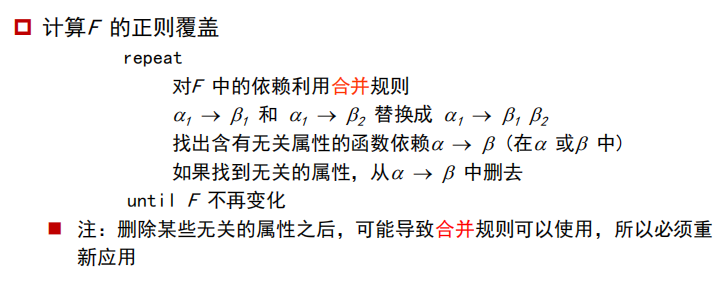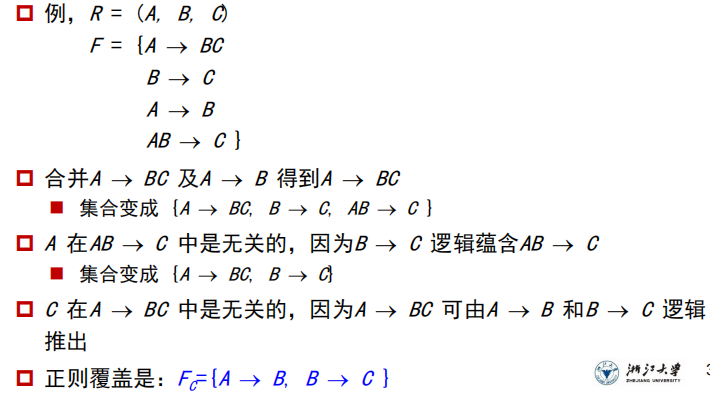规范化-模式分解
当R 不是“好的”形式时，将它分解成模式集合{R1, R2, …, Rn}使得

每个关系模式都是**“好的”形式**

分解是无损连接分解

分解是保持依赖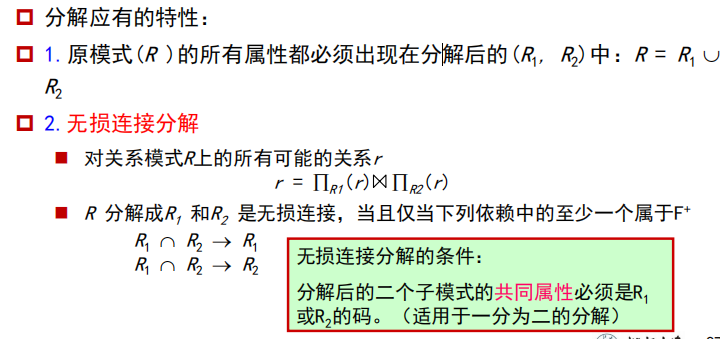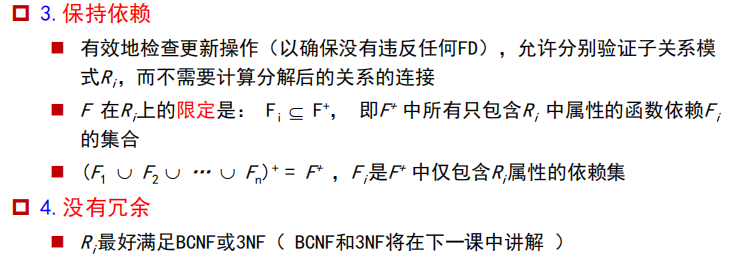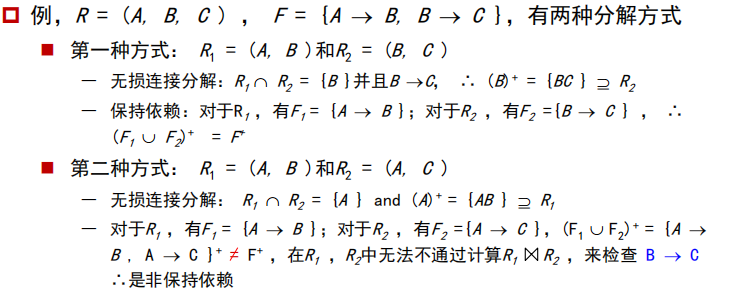分解验证算法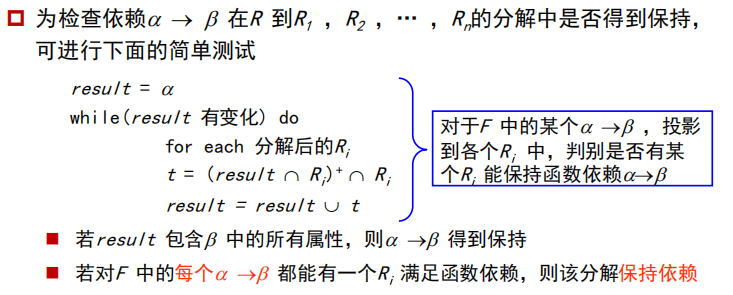模式分解总结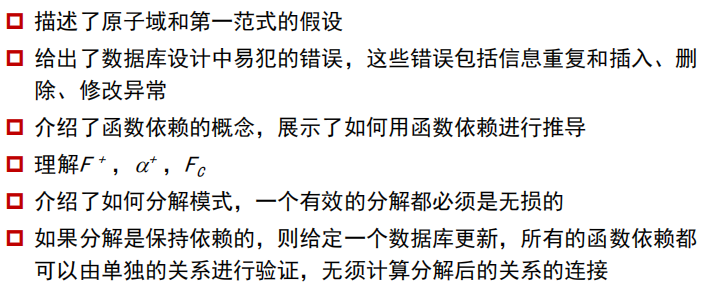规范化-简单版
图示法求候选键
条件：给出函数依赖集，求候选键

将关系的函数依赖关系用有向图表示
找出入度为0的属性，并以该属性集合为起点，尝试遍历所有的图，若能遍历途中所有结点，则该属性集为关系模式的候选键
若入度为0的属性集不能遍历途中所有的结点，则需要尝试性的将 一些中间结点（既有入度，也有出度的结点）并入入度为0的属性集中，直至该集合能遍历所有结点，集合求候选键

主属性和非主属性
组成候选码的属性是主属性、其他的就是非主属性
第一范式（1NF）
在上面
第二范式（2NF）
当且仅当关系模式R是第一范式（1NF），且每一个非主属性完全依赖（A->B->C）候选键（没有不完全依赖（部分依赖（AB->C/A->C））时），则称关系模式R为第二范式
例子：关系模式SC（学号、课程号、成绩、学分），其中（学号，课程号）->成绩，
课程号->学分
分析：因为存在部分依赖，故不满足第二范式
解决：分解成（学号、课程号、成绩）（课程号，学分）
第三范式（3NF）
在2NF上取消完全依赖
例子：
学生关系（学号、姓名、系号、系名、系位置）
分析：该模式存在完全函数依赖（学号->系号–>系名）
解决：分解成（学号、姓名、系号） （系号、系名、系位置）
BC范式（BCNF）
设R是一个关系模式，F是它的依赖集，R属于BCND当且仅当其F中每个依赖的决定因素必定包含R的某个候选码（取消主属性对候选键的部分和传递依赖）
模式分解-保持函数依赖分解
原则：两个关系模式之间不能乱推，得看依赖集
模式分解-无损分解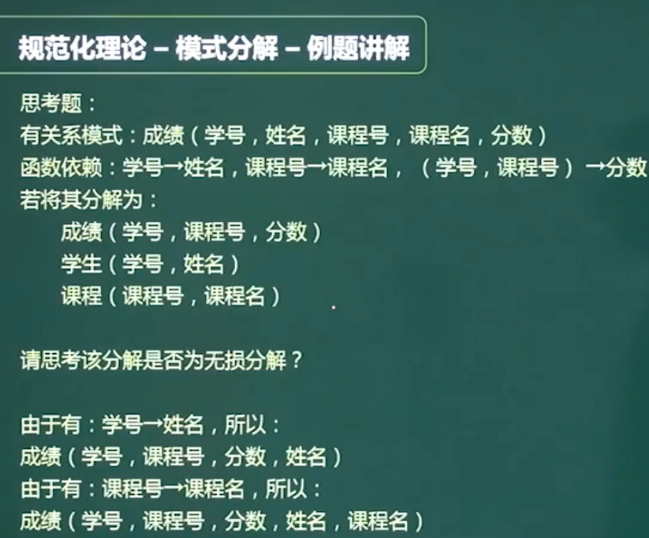展开全文数据库 系统原理
• 文章目录一，第一范式关系数据库设计中易犯的错误数据冗余插入、删除、修改异常模式分解函数依赖（FD）函数依赖的使用函数依赖集的闭包Armstrong 公理计算 F^+^属性集的闭包属性闭包的用途正则覆盖无关属性检测属性...数据库 mysql
• 递归的python函数，用于分解由多个单词组合而成的主题标签或复合单词 我在自然语言处理（NLP）中使用的最大匹配算法的实现，用于将复合词或主题标签拆分为多个词。 用法示例： >>> split_hashtag_to_words_all_...Python
• §2.1 导数的概念 一、从两个例子来谈导数的概念 ...设动点于时刻在直线上所处的位置为，于是，称此函数为位置函数。该如何定义动点在某一时刻的瞬时速度呢? 考虑从到这一时间间隔，质点从位置移动到，其质高等数学 导数与微分 求导法则
• 分解CString的函数AfxExtractSubString 2009年08月01日 星期六 20:46 用AfxExtractSubString()解析复合串如下： CString str = "123,456,789"; CString output = ""; for (int i=0; i<3; i++) { AfxExtractSub.....
• 现在借此机会来重温复习一下。(简要说明一下，因为字符的关系，在本文里 ∈有时代表”属于“，有时代表”真包含“的意思，不便之处，敬请谅解） 函数依赖 函数依赖是一个从数学理论中派生的术语，它指明属性中的每...
• 关系数据库设计理论的核心是数据间的函数依赖，衡量的标准是关系规范化的程度及分解的无损连接性保持函 数依赖性。 数据依赖是通过一个关系中属性间值的相同与否体现出来的数据间的相互关系 函数依赖（FD）是...
• 一、函数依赖：在关系R中，若属性或者属性集 A 中 两个元祖的值相等，如果这两个元祖中对应的属性或者属性集B中的值也相同，则记作A——>B。 A函数决定B； 或者 B函数依赖于A。例1：...
• 一、函数依赖：在关系R中，若属性或者属性集 A 中 两个元祖的值相等，如果这两个元祖中对应的属性或者属性集B中的值也相同，则记作A——&gt;B。 A函数决定B； 或者 B函数依赖于A。例1：下表就是问题领域, 则存在...数据库 mysql sql
• 经贝叶斯推断得到基于</span><span xss=removed>L</span><span xss=removed>2,1</span><span xss=removed>正则化的联合稀疏贝叶斯解混模型，并将丰度向量的非负与为一约束加入到凸优化的目标函数中，通过变量分离...
• ## 函数

2011-05-16 20:36:00
函数 　在第一章中已经介绍过，Ｃ源程序是由函数组成的。 虽然在前面各章的程序中都只有一个主函数main()， 但实用程序往往由多个函数组成。函数是Ｃ源程序的基本模块， 通过对函数模块的调用实现特定的功能...存储 语言 float c
•  数学形态学是一门建立在集论基础上的学科，是几何形态学分析描述的有力工具。数学形态学的历史可回溯到19世纪。1964年法国的MatheronSerra在积分几何的研究成果上，将数学形态学引入图像处理领域，并研制了...
• 【牛客网数据库原理题目】设关系模式R(A，B，C)，F是R上成立的FD集，F={A→B，C→B}，ρ={AB，AC}是R的一个分解，那么分解ρ( )？ 正确答案：C你的答案：A（错误） ( A ) 保持函数依赖集F ( B ) 丢失了A→B ( C ) ...
• 分为无参函数、有参函数和函数。在定义函数时定义形参，调用函数时传递实参；函数返回值通过return获得；不带返回值时定义为空类型。函数调用形式为函数名 (实参表列)。C语言可以嵌套调用函数。在调用函数时又调用...C语言入门
• compose函数–计算复合函数 colspace函数–计算列空间的基 symsum函数–计算表达式的 collect函数–合并同类项 expand函数–展开符号表达式 factor函数–符号因式分解 simplify函数–化简符号表达式 numden函数–...
• 内部数学常数 2 基本数学运算符 3 关系运算符 4 常用内部...进行函数复合运算 7 因式分解 8 代数式展开 9 合并同类项 10 进行数学式化简 11 进行变量替换 12 进行数学式的转换 调用Maple中数学式的转换命令,调用格式
• 文章目录Python函数和函数式编程1、函数概述1.1函数的基本概念1.2函数的功能1.3Python中函数的分类2、函数的声明调用2.1函 数对象的创建2.2函数的调用2.3函数的副作用3、参数的传递3.1形式参数实际参数3.2形式...
• 一、函数依赖概念    函数依赖是从数学角度来定义的，在关系中用来刻画关系各属性之间相互制约而又相互依赖的情况。函数依赖普遍存在于现实生活中，比如，描述一个学生的关系，... 此时， 就可以称“姓名”数据库 存储 insert delete
• 1,sql慢查询 在配置文件中加上long_query_time=2;...优化 使索引起作用 优化数据库结构 分解关联查询 优化分页 将筛选字段加上索引 关联延迟 建立复合索引 脏读 不可重复读 幻读 读未提交 V V V 读已提交 X V ...
• 什么是函数1.1 逆函数（Inverse function）1.2偏函数（Partial function）1.3复合函数（Compound function）1.4多参函数（multi-parameter function）1.5函数柯里化（currying）1.6偏应用函数偏函数 vs 部分应用函数...Java...